Practice the AP 7th Class Maths Bits with Answers Chapter 5 Triangle and Its Properties on a regular basis so that you can attempt exams with utmost confidence.

## AP State Syllabus 7th Class Maths Bits 5th Lesson Triangle and Its Properties with Answers

Question 1.
In the figure x° =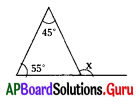A) 45°
B) 55°
C) 100°
D) 80°
C) 100°

Question 2.
In the figure x°, y° are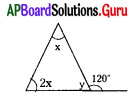A) 60°, 120°
B) 40°, 60°
C) 80°, 60°
D) 180°, 60°
B) 40°, 60°Question 3.
In the figure y° = ……………..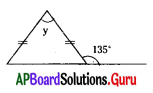A) 45°
B) 55°
C) 90°
D) 35°
C) 90°

Question 4.
If one acute angle of a right-angled triangle is 20°, the other angle is ……………..
A) 70°
B) 20°
C) 60°
D)80°
A) 70°

Question 5.
In an isosceles triangle, the non-equal angle (verticele angle) is 40° then equal angles are
A) 60°, 60°
B) 80°, 80°
C) 140°, 140°
D) 70°, 70°
D) 70°, 70°

Question 6.
From the given figure ⌊x = ………….?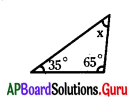A) 60°
B) 90°
C) 70°
D) 80°
D) 80°

Question 7.
From the given figure ⌊y = …………..?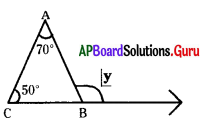A) 130°
B) 60°
C) 20°
D) 120°
D) 120°

Question 8.
From the given figure ⌊x – ⌊y = ?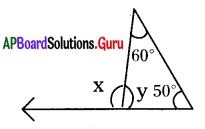A) 70°
B) 20°
C) 40°
D) 50°
C) 40°

Question 9.
The exterior angle of an equilateral triangle is ……………..?
A) 60°
B) 90°
C) 120°
D) 30°
C) 120°Question 10.
If the measure of two angles of a triangle are 35°, 84°, then the third angle is ……………..?
A) 51°
B) 61°
C) 69°
D) 59°
B) 61°

Question 11.
Three angles of a triangle are (x + 30°), 2x, (3x – 30°) then ⌊x = ……………..?
A) 30°
B) 60°
C) 20°
D) 15°
A) 30°

Question 12.
In a right angled triangle one is 43° then the third angle is ……………….
A) 47°
B) 45°
C) 57°
D) 37°
A) 47°

Question 13.
From the given figure ⌊y = …………….?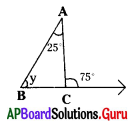A) 40°
B) 50°
C) 60°
D) 75°
B) 50°

Question 14.
In Δ ABC AB = AC then ⌊B = ……………..?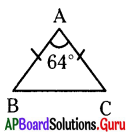A) 58°
B) 68°
C) 48°
D) 116°
A) 58°

Question 15.
The exterior angle of atriangle is 136° and its two opposite interior angles is 8 : 9 then the smallest angle of these two angles is …………….?
A) 54°
B) 72°
C) 64°
D) 66°
C) 64°

Question 16.
Which of the following is an Isosceles triangle?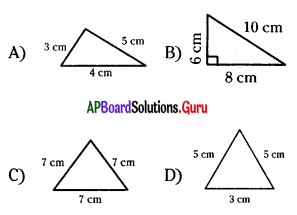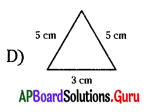Question 17.
Which of the following is an Right angled Isosceles triangle?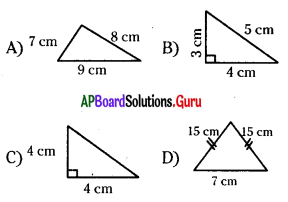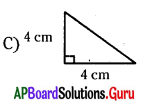Question 18.
Which of the following is not true?
A) A triangle has 3 sides, 3 angles and 3 vertices
B) The angle which is opposite to xy is ⌊Z in ΔXYZ
C) The side which is opposite to ⌊Q is $$\overline{\mathrm{QR}}$$
D) The vertex which is opposite to YZ is X.
C) The side which is opposite to ⌊Q is $$\overline{\mathrm{QR}}$$

Question 19.
Which of the following measurements form a triangle?
A) 4 cm, 4 cm, 8 cm
B) 5 cm, 6 cm, 11 cm
C) 7 cm, 18 cm, 4 cm
D) 7 cm, 8 cm, 9 cm
D) 7 cm, 8 cm, 9 cm

Question 20.
About a triangle which of the following is true?
A) be formed with collinear points
B) have more than one right angle
C) have two obtuse angles
D) have at least two acute angles
D) have at least two acute angles

Question 21.
A triangle can have …………….. right angles.
A) 1
B) 2
C) 3
D) 0
A) 1

Question 22.
A triangle can have …………….. obtuse angles.
A) 0
B) 1
C) 2
D) 3
B) 1

Question 23.
The line joining a vertex and the mid point of its opposite side is called
A) side
B) altitude
C) height
D) median
D) medianQuestion 24.
The perpendicular line segment joining a vertex and its opposite side is called
A) altitude
B) median
C) bisector
D) none
A) altitude

Question 25.
In the figure ΔABC is a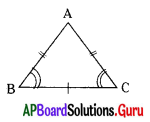A) equilateral
B) isosceles
C) scalene
D) right isosceles B
B) isosceles

Question 26.
A triangle in which all the sides / angles are equal is called ……………. triangle.
A) an equilateral
B) an isosceles
C) right angled
D) scalene
A) an equilateral

Question 27.
A triangle in which one angle is 90°, other two sides are equal is called …………… triangle.
A) Right angled
B) Right angled Isosceles
C) Isosceles
D) Equilateral
B) Right angled Isosceles

Question 28.
A triangle in which each angle is less than 90° is called …………. triangle.
A) right angled
B) an obtuse angled
C) an acute angled
D) None
C) an acute angled

Question 29.
A triangle in which one angle is greater than 90° is called ……………… angled triangle.
A) an Acute
B) right
C) an Obtuse
D) None
C) an Obtuse

Question 30.
The point of concurrence of Medians of a triangle is called ……………
A) Circumcentre
B) Centroid
C) Orthocentre
D) Incentre
B) CentroidQuestion 31.
The altitude of an obtuse angled triangle lies …………….. of the triangle.
A) inside
B) outside
C) on the triangle
D) none
B) outside

Question 32.
The sum of any two sides of a triangle must be ……………… the third side.
A) greater than
B) equal to
C) less than
D) none